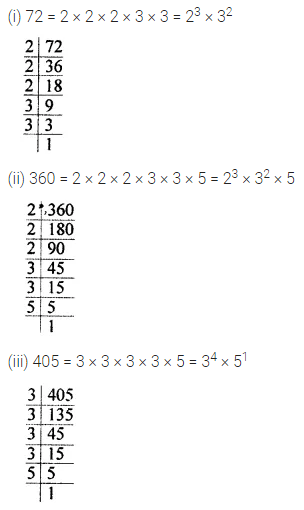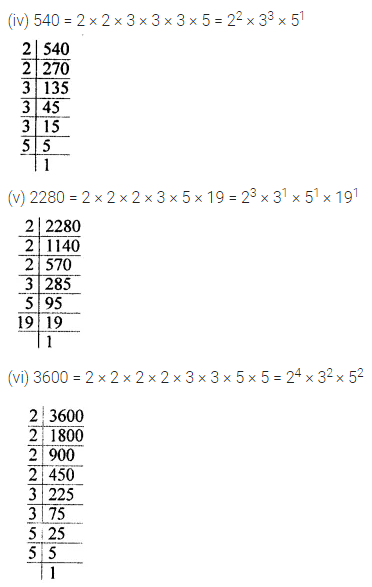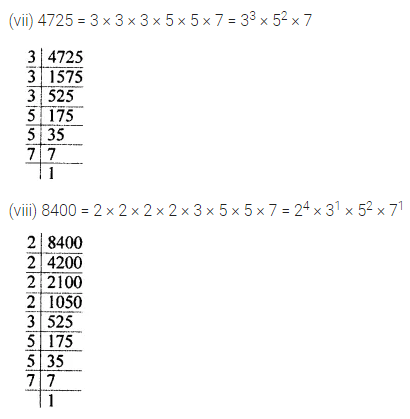# ML Aggarwal Class 7 Solutions for ICSE Maths Chapter 4 Exponents and Powers Ex 4.1

## ML Aggarwal Class 7 Solutions for ICSE Maths Chapter 4 Exponents and Powers Ex 4.1

Question 1.
Fill in the blanks:
(i) In the expression 37, base = …….. and exponent = ……..
(ii) In the expression (-7)5, base = ………. and exponent = …….
(iii) In the expression $$\left( \frac { 2 }{ 5 } \right) ^{ 11 }$$, base = …… and exponent = …….
(iv) If base is 6 and exponent is 8, then exponential form = ……..
Solution: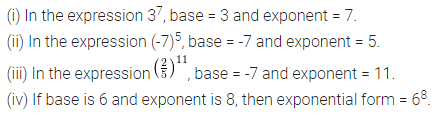Question 2.
Find the value of the following:
(i) 26
(ii) 55
(v) (-6)4
(vi) $$\left( \frac { 2 }{ 3 } \right) ^{ 4 }$$
(v) $$\left( \frac { -2 }{ 3 } \right) ^{ 5 }$$
(vi) (-2)9
Solution: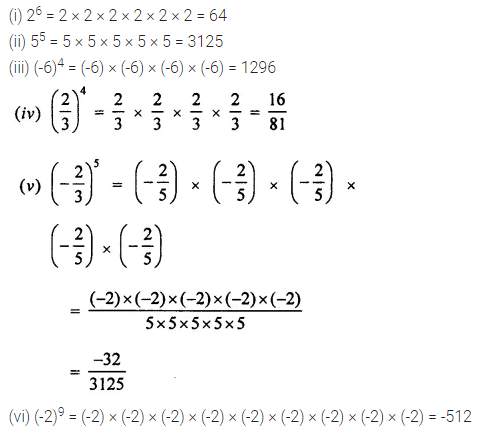Question 3.
Express the following in the exponential form:
(i) 6 × 6 × 6 × 6 × 6
(ii) t × t × t
(iii) 2 × 2 × a × a × a × a
(iv) a × a × a × c × c × c × c × d
Solution:Question 4.
Simplify the following:
(i) 7 × 103
(ii) 25 × 9
(iii) 33 × 104
Solution: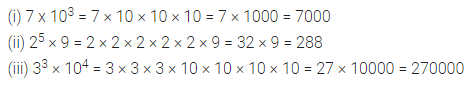Question 5.
Simplify the following:
(i) (-3) × (-2)3
(ii) (-3)2 × (-5)2
(iii) (-2)3 × (-10)4
(iv) (-1)9
(v) 252 × (-1)31
(vi) 42 × 33 × (-1)122
Solution:Question 6.
Identify the greater number in each of the following:
(i) 43 or 34
(ii) 73 or 37
(iii) 45 or 54
(iv) 210 or 102
Solution:Question 7.
Write the following numbers as powers of 2:
(i) 8
(ii) 128
(iii) 1024
Solution: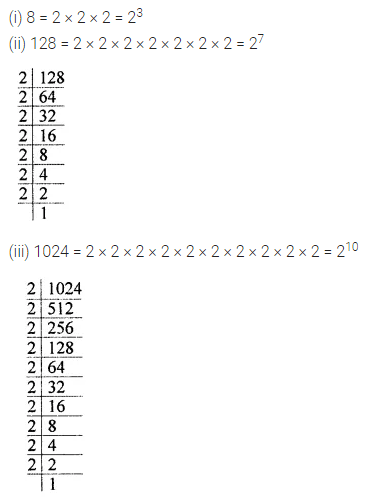Question 8.
To what power (-2) should be raised to get 16?
Solution: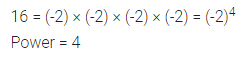Question 9.
Write the following numbers as powers of (-3):
(i) 9
(ii) -27
(iii) 81
Solution:Question 10.
Find the value of x in each of the following:
(i) 7x = 343
(ii) 3x = 729
(in) (-8)x = -512
(iv) (-4)x = -1024
(v) $$\left( \frac { 2 }{ 5 } \right) ^{ x }$$ = $$\frac { 32 }{ 3125 }$$
(vi) $$\left( \frac { -3 }{ 4 } \right) ^{ x }$$ = $$\frac { -243 }{ 1024 }$$
Solution: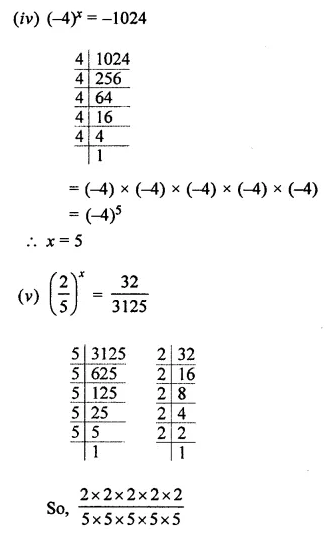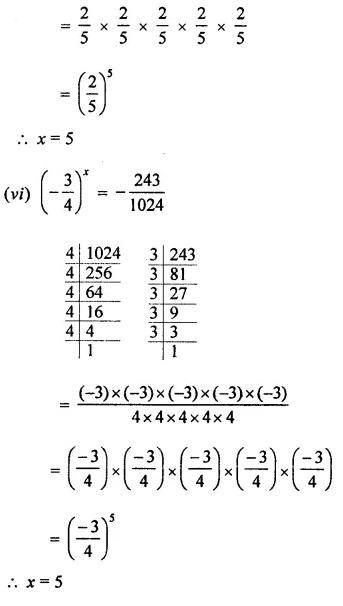Question 11.
Write the prime factorization of the following numbers in the exponential form:
(i) 72
(ii) 360
(iii) 405
(iv) 540
(v) 2280
(vi) 3600
(vii) 4725
(viii) 8400
Solution: